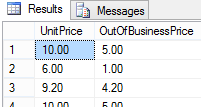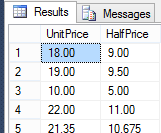Tech Junkie Blog - Real World Tutorials, Happy Coding!: SQL: Mathematical Operators + , - , * , /

## Monday, March 2, 2015

SQL: Mathematical Operators + , - , * , /

As you may have guessed the SQL mathematical operators are equivalent to their regular mathematical counter parts.

2. - Subtraction
3.  * Multiplication
4.  / Division
Eamples:

```SELECT UnitPrice, (UnitPrice + 20) AS RipOffPrice
FROM Products
```2. Subraction

```SELECT UnitPrice,(UnitPrice - 5) AS OutOfBusinessPrice
FROM Products
WHERE UnitPrice BETWEEN 5 AND 10
```3. Muliplication

```SELECT UnitPrice,(UnitPrice*UnitsInStock) AS InventoryPrice
FROM Products
```4. Division

```SELECT UnitPrice,(UnitPrice/2) AS HalfPrice
FROM Products
```#### 1 comment:

1.